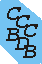Computational Chemistry Comparison and Benchmark DataBase Release 22 (May 2022) Standard Reference Database 101 National Institute of Standards and Technology Home All data for one species Geometry Experimental Calculated Comparisons Bad Calculations Tutorials and Explanations Vibrations Experimental Calculated Scale factors Reactions Entropies Ions List Ions Energy Electron Affinity Proton Affinity Ionization changes point group Experimental One molecule all properties One property a few molecules Geometry Vibrations Energy Electrostatics Reference Data Calculated Energy Optimized Reaction Internal Rotation Orbital Nuclear repulsion energy Correlation Ion Excited State Basis Set Extrapolation Geometry Vibrations Frequencies Zero point energy (ZPE) Scale Factors Bad Calculations Electrostatics Charges Dipole Quadrupole Polarizability Spin Entropy and Heat Capacity Reaction Lookup by property Comparisons Geometry Vibrations Energy Entropy Electrostatics Ion Resources Info on Results Calculations Done Basis functions used I/O files Glossary Conversion Forms Links NIST Links External links Thermochemistry Tutorials Vibrations Entropy Energy Electrostatics Geometry Cost Bad Calculations FAQ Help Units Choose Units Explanations Credits Just show me Summary Using List Recent molecules Molecules Geometry Vibrations Energy Similar molecules Ions, Dipoles, etc. Index of CCCBDB Feedback You are here: FAQ Help > Units > Explanations > Hartree

# What's a hartree?

A hartree is a unit of energy used in molecular orbital calculations. A hartree is equal to 2625.5 kJ/mol, 627.5 kcal/mol, 27.211 eV, and 219474.6 cm-1.

The energy reported from a calculation is the energy for the separation of the molecule to nuclei and electrons. For example the HF/6-31G* energy for CH4 is -40.195172 hartrees, which refers to the energy for the reaction CH4 => C6+ + 4 H+ + 10 e-

For semiempirical calculations (AM1, PM3) the energy is reported in hatrees, but the energy refers to the heat of formation for that species at 298 K. For example the PM3 energy for CH4 is -0.020766 hartrees which can be converted directly to a heat of formation of (-0.020766 hartrees)(2625.5 kJ/mol/hartree) = -54.5 kJ/mol.

More on units and conversions can be found near the end of the page discussing thermochemistry (Resources; Thermochemistry; Thermochemistry)